# MCQs on Electric Circuits

##### Page 47 of 63. Go to page 1 2 3 4 5 6 7 8 9 10 11 12 13 14 15 16 17 18 19 20 21 22 23 24 25 26 27 28 29 30 31 32 33 34 35 36 37 38 39 40 41 42 43 44 45 46 47 48 49 50 51 52 53 54 55 56 57 58 59 60 61 62 63
01․ The energy required to charge a 10 μF capacitor to 100 V is
0.01 J.
0.05 J.
5 × 10-9 J.
10 × 10-9 J.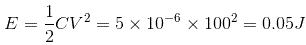02․ R1 = 36 Ω and R2 = 75 Ω, each having tolerance of ±5% are connected in series. The value of resultant resistance is
111 ± 0 Ω.
111 ± 2.77 Ω.
111 ± 5.55 Ω.
111 ± 7.23 Ω.

R1 = 36 ± 5% = 36 ± 1.8 Ω. R2 = 75 ± 5 % =75 ± 3.75 Ω. ∴ R1 + R2 = 111 ± 5.55 Ω.

03․ A particular current is made up of two component : a 10 A and a sine wave of peak value 14.14 A. The average value of current is
zero.
24.14 A.
10 A.
14.14 A.

Average DC current = 10 A. Average AC current = 0 A as it is alternating in nature. Average current = 10 + 0 = 10 A.

04․ In the circuit given below the value of resistance Req is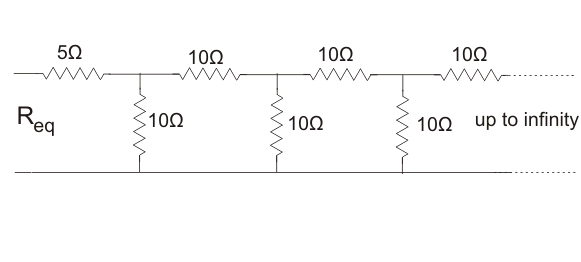10 Ω.
11.86 Ω.
11.18 Ω.
25 Ω.

The circuit is as shown in figure below.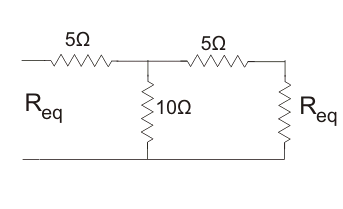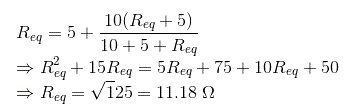05․ The switch S is the circuit shown in figure is ideal. If the switch is repeatedly closed for 1 ms and opened for 1 ms, the average value of i(t) is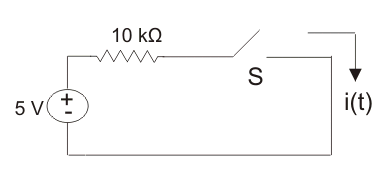0.25 mA.
0.35 mA.
0.5 mA.
1 mA.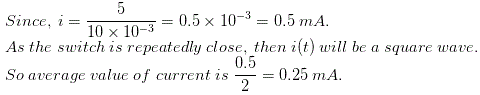06․ The linear circuit element is
capacitor.
inductor.
resistor.
all of above.

A linear circuit element does not change their value with voltage or current.

07․ Active element of electrical circuit is
voltage source.
current source.
both of them.
none of above.

Active elements are used to drive the circuit. They cause the current to flow through the circuit or the voltage drop across the element.

08․ The basic circuit elements are
R, L and C.
Vs, Is.
both A and B.
none of the above.

The elements, which show their behavior only when they are excited, are called basic circuit element.

09․ The resistance of a strip of copper of rectangular cross section is 2 Ω. A metal of resistivity twice that of a copper is coated on its upper surface to a thickness equal to that of copper strip. The resistance of composite strip will be
3 / 4 Ω.
4 / 3 Ω.
3 / 2 Ω.
6 Ω.

Copper and coated metal strip have resistance 2 ohms respectively. These two strips are in parallel.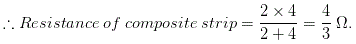10․ The form factor of sinusoidal alternating current is
0.
1.
1.11.
1.15.

For alternating current form factor is defined as the ratio of rms value and average value of alternating current. Now the rms value of alternating current = 0.07 × maximum value of alternating current. Average value of alternating current = 0.637 × maximum value of alternating current.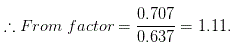<<<4546474849>>>